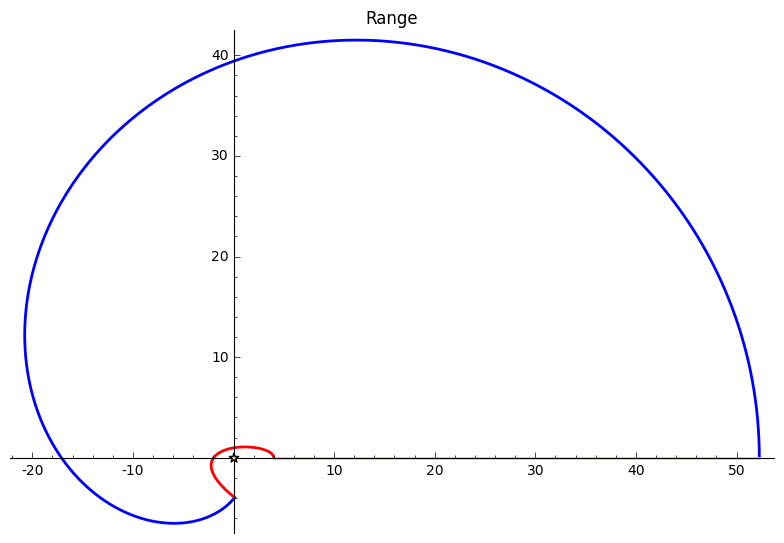# The Argument PrincipleThis post illustrates the Argument Principle.

Let \$f\$ be a polynomial, and \$C\$ be (an arc of) a circle of radius \$R\$ centered at the origin.

The code below generates 2 plots. The first plot shows the domain of \$f\$. We plot the roots of \$f\$ together with \$C\$. Let \$n_1\$ be the number of roots contained within \$C\$.

The second plot shows the range of \$f\$. We plot \$f(C)\$, along with a marker at the origin. Let \$n_2\$ be the number of times the curve winds around the origin.

You can verify that \$n_1 = n_2\$. As you vary the radius \$R\$, observe how \$C\$ and \$f(C)\$ change, and how this affects \$n_1\$ and \$n_2\$.

Written on November 19, 2014